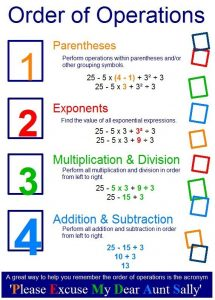# Handouts

Regents Formula Sheet

Algebra 1 Midterm Review Packet

Algebra 2 Midterm Review 17 pages

Algebra2 Midterm Practice Questions 2018

Algebra Formulas

Calculus Formulas

Common_Derivatives_Integrals

Converting-Words-to-Mathematics

Exponent-Rules

Geometry Formulas

Geometry Midterm Review

Math Formulas 2

Properties

Rules for Working with Signed Numbers

Trigonometry Formulas

Math isn’t always about calculations.

Often it is about recognizing patterns,

attempting to understand those patterns,

and explaining what those patterns mean.